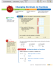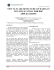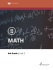Probability Lesson 3 â Video Notes Guide Independent Events By

Transcription

Probability Lesson 3 â Video Notes Guide Independent Events By
Math 7
Unit – Probability
Lesson 3 – Video Notes Guide
Independent Events
By the end of this lesson you will be able to ____________________________________________________.
What is Probability?______________________________________________________________________.
What three ways can probability can be represented?
1) _____________________ 2) ______________________ 3) ____________________
What values represent Certain?
_______
_______
_______
What values represent As Likely As Not?
_______
_______
_______
What values represent Impossible?
_______
_______
_______
What are independent events? ______________________________________________________________.
How is rolling a die an independent event?
How is flipping a coin an independent event?
Example 1: Using an Area Model
What is the probability that you can roll a ______ on a die and flip ________ up on a coin?
Outcomes for
the 2nd event
What is the ratio we use for probability?
____________________________________________
Outcomes for
the 1st event
How many outcomes do the two events
have together?
What is the probability of the events
occurring together?
As a fraction? ____________________
As a decimal? ____________________
As a percent? ____________________
Example 2: Using Multiplication
What is the probability that you can flip ___________ up on a coin and roll a _______ on a 12-sided
die?
Outcomes for
the 2nd event
Outcomes for
the 1st event
What is the probability for the first event to occur? ____________ 2nd Event to occur? ___________
What operation will you use to determine the probability of these events occurring together? ________
What is the probability for these events to occur together?
As a fraction? _________
As a decimal? _________
As a percent? ________
Example 3: With Replacement
What is the probability that you can pick a red card put it back in the deck and pick the black
card?
What is the probability for the first event to occur? ____________ 2nd Event to occur? ___________
What operation will you use to determine the probability of these events occurring together? ________
What is the probability for these events to occur together?
As a fraction? _________
As a decimal? _________
As a percent? ________
Example 4: With Replacement
What is the probability that you can pick the Ace of Hearts, put it back in the deck, and pick the King of
Clubs?
What is the probability for the first event to occur? ____________ 2nd Event to occur? ___________
What operation will you use to determine the probability of these events occurring together? ________
What is the probability for these events to occur together?
As a fraction? _________
Math 7
As a decimal? _________
Unit – Probability
As a percent? ________
Your Turn to Practice. [fill in the missing information for each problem using the video]
Find the probability of the events. Write as a fraction, decimal and a percent. Round to the nearest
thousandths when necessary.
1) Probability of rolling an _______ number on a standard die and flipping a _________.
2) Probability of choosing two vowels, with replacement, from the word ___________________.
3) Probability of picking an _______ number, putting it back, and then picking a multiple of _____,
from the numbers ____________.
4) Probability of rolling a die three times, all coming up _________ numbers.
5) Probability of picking a red gumball, replace it and then choose a blue gumball, from ______ red
and ______ blue gumballs.

Unit 13.3A.notebook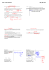ROSE X-Lite Team-8800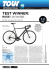Math Wuzzles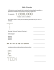…convert fractions, percentages and decimals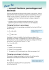Percent decimals and fractions.notebook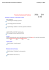Dewey Decimal Classification System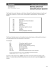Changing a Percent to a Fraction or a Decimal 6.1 OBJECTIVES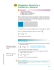Year 9 Higher â Number revision Use this revision list ALONGSIDE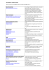Changing Decimals to Fractions Now